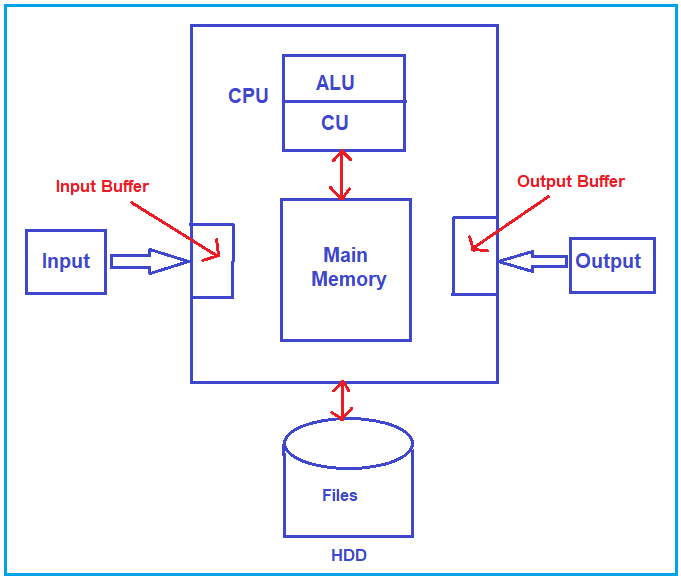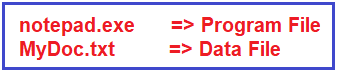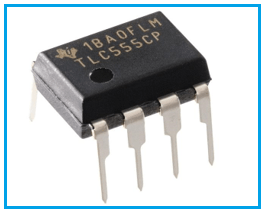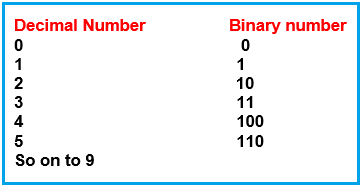# How Computer Works

## How Does the Computer Work?

In this section we will be discussing some fundamentals before learning a programming language get awareness about a few things if you are already aware of this then you can skip the section and you can go to the next section. Mostly in academics, these are the basics that are taught. So, to get the awareness about the computers so that everyone can understand how to use the computer means how to write the programs. How do you utilize the best? Following are the pointers we will be discussing in this article.

1. How does the computer work?
2. Number system.
3. What is a Program?
4. Low-level and High-level Language.
5. Computer and Interpreters.
6. Operating System.
##### How Does the Computer Work?

In this article, I am going to discuss how computers work. To understand how computer works, please have a look at the following basic block diagram of a computer and understand the different components of a computer.Let’s study the components of a computer. The computer consists of the following components.

1. CPU- Central Processing Unit.
2. Main Memory
3. Hard disk
4. Input devices
5. Output devices

Let us understand the components in detail.

##### CPU

CPU is the heart of the computer. CPU stands for central processing unit or microprocessor. The Brain of the computer is the CPU where actual data manipulation happens using ALU (Arithmetic and logical unit). If you see the present-day processors like i3, i5, i7, etc., these are processors. The CPU is consisting of two more components i.e. ALU (Arithmetic Logic Unit) and CU (Control Unit).

1. ALU- Arithmetic Logic Unit: ALU performs the basic logical and arithmetic operations. Arithmetic operations like addition, subtraction, multiplication, division, and modulus. Logical operations like comparing two numbers (such as >, <, >=, <=, etc.), logical AND, OR, NOT, etc. So, ALU has performed these basic operations.
2. CU- Control Unit: Control unit controls the other components like Input and output devices, main memory, hard disk, etc. The job of the control unit is to utilize other resources.
##### Hard Disk:

A hard disk is the storage device for a computer. It contains two types of files, i.e. data files and program files. We can store data or programs on the hard disk and it is permanent storage.

If you take an example, the notepad on your computer is a program file. So, when you open notepad it’s a program and there is a file available. So, basically, we click on the notepad icon and the program will start, but if you look into My Computer and folders, somewhere you will find the program file for the notepad and the file name will be notepad.exe. Now, if you type something on the notepad, and save it, then it will save as a text file such as MyDoc.txt and it is a data file and this data file is used by notepad or generated by notepad. For better understanding, please have a look at the below diagram.Program files: The music player in the computer or notepad in the computer are program files. Any application or software installed on the computer

Data files: Movies, songs, word documents, etc. are types of data files. It contains actual data, writes/reads into the data we actually need a program file.

##### Main Memory:

Whenever the user wants to run any program, then it should be present in the main memory so that the CPU can execute it. The program is brought from the Hard Disk into the Main Memory so that it can be executed by the CPU. This is the reason why Main memory is also called working memory or Primary Memory.

Once the program is brought to the Main memory, the only CPU executes the program line by line. So, whatever program we run, they are brought into the Main memory. If the program needs some data from the keyboard or if it has to display some data on the monitor, then it can utilize the INPUT and OUTPUT devices.

##### Input Devices:

Input devices are keyboard, mouse, etc. These devices are used to give inputs to the computer. Input devices have their own memory in the computer called Input Buffer. All instructions from input devices are dumped into this memory and if the program wants to read something, then it will read from the input buffer. So, whatever we type using the keyboard will be stored in the input buffer and the program reads the input from the input buffer.

##### Output Devices:

Output devices are monitors, printers, etc. Output devices show the output after the execution of the program file. Output devices also have their own memory called output buffer. If the program wants to print something, then whatever it wants to print will be put in the output buffer and that will appear in the output screen.

Above are the components of a computer system and this is how a program works inside it. You might have one question in your mind about how a computer understands the programming language or instructions. So, let’s look at it. The computer only understands the binary number system.

The computer thinks only in Binary 0’s and 1’s because the whole computer is a circuit. Built from gates (logic gates). So, we can say computers operate only on Boolean algebra. Any Computational /Algebraic or logical task that the computer performs receives data in the form of 0’s and 1’s and manipulates the data using logic gates.

##### What is a binary number system?

Computers are electronic machines and any electronic machines works on voltage and current. Voltages are logically used as binary. Low-level voltage is treated as 0 and high-level voltage is treated as 1.

Consider you have a bulb, so there are two possibilities either bulb is on or off. So, when the bulb is on, we consider it as 1 and when it is off, we consider it as 0. So, we can achieve the binary language using the voltage.

If you have Seen any Electronic Circuit and it is having zeroes or ones, means on and off. So, one bulb can give us 2 messages i.e. On or Off or high voltage or low voltage. If we increase the number of bulbs to two, we will get 4 different messages. As we increase the number of bulbs it will give us more instructions or patterns.

2- bulbs – 2^2= 4 instructions
4 bulbs – 2^4 =16 instructions
.
.
.
16 bulbs – 2^16= 65536 instructions.

Following is an image of electronic circuitry i.e. IC (integrated circuit) and also called a chip. The ICs are used to perform some tasks and work on a binary number system.The above IC is an 8-pin IC. You can consider each pin as a bulb that can store some values or instructions. So, this IC can generate 8 bits of instructions. Which will be further used for executing the tasks. Like this, the binary number system is used to make communication.

So, we learned that the Binary system is used for communication and to pass instructions to computers. But we are aware of the decimal system only. Relation of binary number system with decimal as follows.Using the binary number, the ALU performs the arithmetic and logical operations. For example- ALU has defined a set of commands. When there is 1 perform addition, 2 then perform multiplication, etc. ALU already knows for which command, and what it has to perform. So, to pass these commands to the CPU, they should be present in the form of a binary system in the main memory. From where the CPU can take these instructions.

In the next article, I am going to discuss Number Systems. Here, in this article, I try to explain How a computer works in detail and I hope you enjoy this article. In the next article, I am going to discuss the Binary Number System in detail.